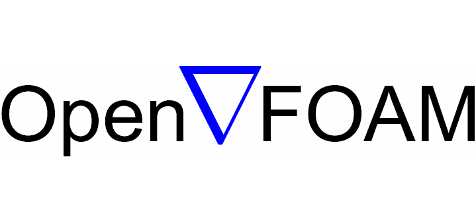# Technology

This part includes technologies, methods and softwares used for our studies; and exposes one example of a study case.

## What does CFD mean?

CFD is the abbreviation of Computational Fluid Dynamics, and is a field of physics that includes all the numerical methods that allow to study a fluid flow (liquids and gases) in a given environment.

All phenomena related to fluid flows are described by a set of four differential equations called “Navier-Stokes” equations. Currently there is no analytical solution of this set and it is one of the seven “Millenium problems” defined by the Clay Mathematical Institute.

Even if there is no analytical solution of those equations, some methods exist to solve it in a finite number of points defined in a given domain under given conditions. All those methods are a field of Mathematics called “Numerical Methods”.

Other equations can be coupled to this original set (Chemical reactions, turbulence, radiation…) in order to solve a very wide range of complex Research & Development problems.

It is also possible :

• to simulate flows inside or around a body,
• to evaluate forces applied on a body exposed to wind or moving in the air (aerodynamics) or in the water (hydrodynamics),
• to determine necessary heating and cooling powers in a building as well as the best places to fit ventilation inlets and outlets,
• to visualize pollutant dispersion, smoke ejection, chemical concentration on different zones of a reactor, corrosion, phase separation (sand and water for example)…

## SoftwaresAll our simulations are performed via ANSYS Fluent ©, market leading software and by far the most complete.

We also develop User Defined Functions (UDF), C programs that are compiled and integrated into Fluent in order to overcome the software limitations for specific applications.DualSPHysics software is based on Smoothed-Particle Hydrodynamics (SPH) method which is a Lagrangian method without any mesh as fluids are modeled as moving particles interacting between each other.

SPH method is really efficient to compute two-phases flow, impacts or explosions, fluid-structure interactions, moving walls or flows in porous media.OpenFoam is a software whose code is open source. It is developed and made available by a wide scientific community.

Its code is editable in order to be adapted to every single case. It allows to realize Multiphysics simulations by solving fluid mechanics equations.

## Study execution

All studies are different but it is possible to define a general methodology applicable to each case. The example given here is the design of a hairdryer diffuser which is a specific and explicite case to illustrate this method.

### Design

The basis of any study is a virtual model of the part to be studied, either from plans, either directly from CAD files. Depending on the case, we are able to simulate only fluids or all fluids and solids present. When the model allows us to do so, we consider plane and axial symmetry to optimize calculation time and thus, realization time. Models are designed with SolidWorks or ANSYS DesignModeler.

### Meshing

At this step, we define nodes where equations will be numerically solved. This involves dividing the volume studied in small elementary volumes called mesh or grid points. The more they are, the more accurate the solution will be but the longer the computation time will be. It is then necessary to define the most interesting areas and then refine the mesh in these areas, such as boundary layers or general areas where one would expect to observe large variations in the different parameters studied. Several meshing methods can be used depending on the model topology. In this case, only two methods are possible, polyhedral mesh and tetrahedral mesh.

### Simulation

Once the mesh achieved, it is necessary to define what are the best numerical methods suited to the problem :

• heat exchange simulation,
• the approach to solve turbulence,
• chemical reaction model,

### Boundary conditions

This is where you define the parameters of the study: wind speed, temperatures of different elements and different fluids, flow rates, pressures, chemical concentrations, etc.

Likewise, all material properties are defined such as density, viscosity, conduction, absorption coefficient for fluids and thermal conductivity, density, emissivity for solids.

### Postprocessing

Postprocessing concerns all results visualization: display of velocity fields, temperature and pressure contours, velocity vectors, streamlines, iso-value contours, numerical values, etc.